# CSVTU Exam Question Papers – BE I Year – Engineering Mechanics–2006-07

BE (1st Year)

Examination-2006-07

Engineering Mechanics

UNIT- I

Q.1 Attempt any two parts :

(a) A smooth cylinder of radius r = 15 cm resting on a horizontal surface support a bar AB of length 45.0 cm which is hinged at A.

The weight of the bar is 100N. The cylinder is kept from rolling away by a string AO (fig. a) of length 30.0 cm.  Assume all surfaces to

be frictionless, find the tension in the string .(b) Determine the forces exerted on the cylinder A and B by spanner wrench due to a vertical ! force of 200 N (fig.2) applied to

the handle Neglect friction at B . Draw free body diagram for cylinder and wrench separately.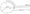(c) A ice cube 300mm is held by means of tongue as shown if fig.3. The dimensions a = 75mm, b = 150, c = 150 mm , d = 300 mm.

If mass of the ice is 10 kg determine the pressure at A and reactions at joint C and D . draw free body diagram of tongue D C B.

UNIT- II

Q.2 Solve any two parts :

(a) Draw shear force and bending moment diagram for over hang beam shown in fig. 4 . calculate maximum bending moment

and position of zero bending moment . Support span AC = 6M.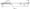(b) Use method of joints and obtain forces in the members of truss shown in fig.5(c) Use method of section and find the forces in members BD , CD and CE of truss shown in fig. 6UNIT- III

Q.3 Solve any two parts

(a) Block A of weight 800 N is to be raised by a wedge R as shown in fig.7. Assume at all surface. Draw force polygon and find the value

of horizontal force P required to raise.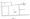(b) Derive a formula for efficiency of square threaded screen jack.

(c) A flat belt of cross sectional area 80mm x 60 mm is running at a speed of 16m/sec . The angle of lap is 160° and the coefficient

of friction = 0.3 . The specific gravity of belt , material is 0.9. Calculate the power transmitted by belt maximum stress in the belt

is not to exceed 200 N/m3 .

The moment of inertia of a body about any two reference directions X and Y are Ix and  and the product of inertia is

. Derive equations to find the magnitude and direction of principle moment of inertia.

Or

For the unequal angle L section shown in fig. 8 obtain the position and magnitude of principle moment of inertia.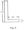UNIT- V

Q.5 Attempt any two parts :

(a) Two blocks of mass M, and A/2 are placed on two inclined planes of inclination 21 and 22 (fig.9). the masses are connected by

a string passing over a smooth pulley. The coefficient of friction : = 0.3  If M1 =15 kg, M2 = 30kg and angle 2, =30°, 22 =60°,find

the acceleration of mass and M1 and M2.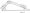(b) A ball of mass M = 5 kg strikes from height h = 10cm over a spring of stiffness K = 5N/cm. Calculate the maximum deflection

of spring.

(c) A guh of mass 500kg fires horizontally a shell of mass 8 kg with a velocity of 20km/hr. What is the velocity which the gun will recoil . Calculate the force required to stop the gun in 0.5 m and the time required to stop it